The user selectable methods for Head Loss include None and Hazen-Williams Equation. None is the default method and makes no other calculations. Hazen-Williams Equation calculates either Inflow Head given Outflow Head, or Outflow Head given Inflow Head. The selected method executes from the dispatch method.None
This is the default method and makes no calculations.Hazen-Williams Equation
This method calculates Inflow Head given Outflow Head (and other inputs) or Outflow Head given Inflow Head (and other inputs). These inputs include pipe diameter, pipe length, the C Value of the Pipeline, and minor losses within the Pipeline.
In this method, either Inflow Head or Outflow Head must be given (either set as an input, by rules, or set through a link) to calculate the other. If Inflow Head is input or set through a link, Outflow Head is calculated. If Outflow Head is input or set through a link, Inflow Head is calculated.
The following slots are instantiated when using the Hazen-Williams Equation method.
Slots Specific to This Method
Type: SeriesSlot
Units: Length
Description: Inflow Head of the Pipeline object. This is equal to the total head, which includes pressure, elevation, and velocity components. This value likely comes from a link or is calculated.
I/O: Input or output
Type: SeriesSlot
Units: Length
Description: Outflow Head of the Pipeline object. This is equal to the total head, which includes pressure, elevation, and velocity components. This value likely comes from a link or is calculated.
I/O: Input or output
Diameter
Type: ScalarSlot
Units: Length
Description: Diameter of the Pipeline. It is assumed constant over its length. It must be greater than zero.
I/O: Input only
Velocity
Type: SeriesSlot
Units: Velocity
Description: Velocity of the water within the Pipeline.
I/O: Output only
Length
Type: ScalarSlot
Units: Length
Description: Length of the Pipeline. The length must be greater than or equal to zero.
I/O: Input only
C Value
Type: ScalarSlot
Units: NoUnits
Description: Hazen-Williams C Value of the Pipeline. The C Value depends on the pipe material, pipe age, tuberculation, lining, and so on. Values can be found in a hydraulics text and vary between 80-150.
I/O: Input only
Minor Losses
Type: ScalarSlot
Units: Length
Description: Minor Losses at the Pipeline. The value defaults to zero if less than zero or not input.
I/O: Input only
Method Details
The head loss is calculated using the Hazen-Williams formula, as follows: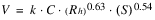Rh is the hydraulic radius. For a circular pipe: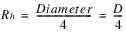V is the velocity of the water within the pipeline, as follows: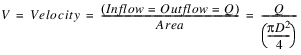C is the user input Hazen-Williams C Value of the pipe, as follows: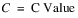S is the slope of the energy grade line, as follows: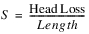Note:  The empirically derived Hazen-Williams formula is unit-specific. Published in literature, k for British units is 1.318 and 0.85 for SI units. In RiverWare, the formula uses S.I. units, in which head loss has units of meters, Length has units of meters, Velocity has units of meters/second, the C Value has no units, and Diameter has units of meters. Unfortunately, k =1.318 for British and k = 0.85 for SI are not equivalent when converted. As a result, RiverWare uses the more common and more significant value of k = 1.318. In SI units, this is equivalent to 0.8492.
Rearranging the Hazen-Williams formula, head loss can be solved as follows: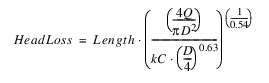Depending on inputs, Outflow Head or Inflow Head is set to one of the following:
•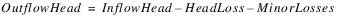•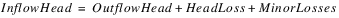Revised: 01/11/2023